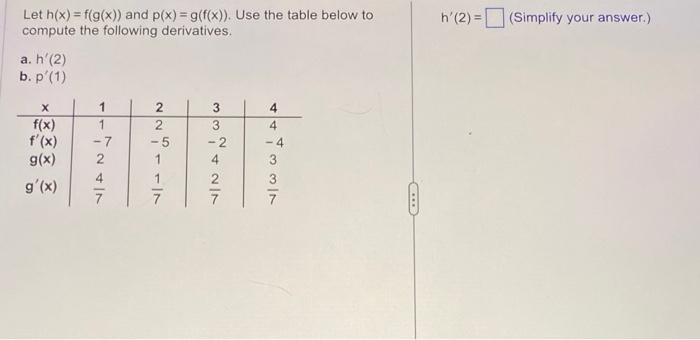Home / Expert Answers / Calculus / a-and-b-let-h-x-f-g-x-and-p-x-g-f-x-use-the-table-below-to-compute-the-following-pa179

# (Solved): a and b Let $$h(x)=f(g(x))$$ and $$p(x)=g(f(x))$$. Use the table below to compute the following ...

a and bLet $$h(x)=f(g(x))$$ and $$p(x)=g(f(x))$$. Use the table below to compute the following derivatives. $$h^{\prime}(2)=$$ (Simplify your answer.) a. $$h^{\prime}(2)$$ b. $$p^{\prime}(1)$$

We have an Answer from Expert